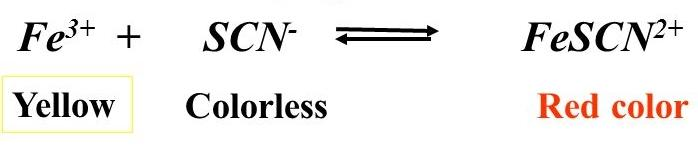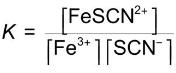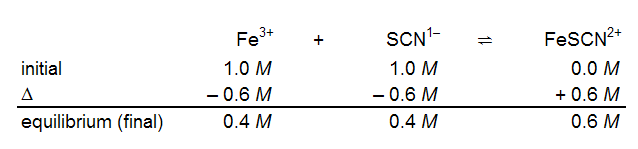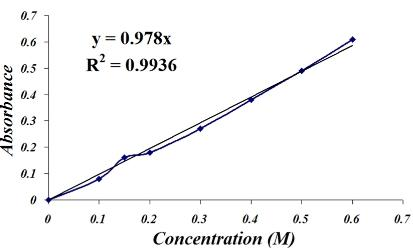# Colorimetric Determination of an Equilibrium Constant in an Aqueous Solution Flashcards

Set Details Share
created 7 years ago by brianquintero1
3,641 views
updated 7 years ago by brianquintero1
Page to share:
Embed this setcancel
COPY
code changes based on your size selection
Size:
X

1

Purpose of this experiment

become familiar w/ the concept of equilibrium by determination of an equilibrium constant

for a reaction in a solution

2

Characteristic of all chemical equilibria

dynamic and constantly proceeding in both directions

3

State of equilibrium

when the rate of the reaction in the forward direction is equal to the rate of the reaction in the reverse direction

forward rate of reaction = reverse rate of reaction

4

What will we use as a source of Fe3+ ions? SCN- ions?

iron (III) nitrate, Fe(NO3)3

sodium thiocyanate, NaSCN

5

Why will we use 0.10M nitric acid instead of water?

Because equilibrium constants are dependent on the total ionic concentration of the equilibrium mixture. By using nitric acid we ensure that all mixtures have comparable ionic concentrations.

equilibrium constants are also temperature dependent, so we'll record the temp at which our measurements are made

6

What is the reaction for the formation of FeNCS2?Fe3+(aq) + SCN-(aq) <--> FeSCN2+

Pale yellow colorless blood red

*Note: this is the simplified version of the equation. The Fe3+ is in reality [Fe(H2O)6]3+ and FeNCS2+ is actually [Fe(SCN)(H2O)5]2+

7

Equilibrium constant expression for the formation of FeNCS2+?Keq= [FeSCN2+]/ [Fe3+][SCN-]

8

Example of the calculation for equilibrium constant (Keq) expression for the formation of FeNCS2+.9

Example of ICE table used for FeNCS2+10

What are the five components of a spectrophotometer?

1. light source producing light w/ a wavelength from about 375-650 nm

2. a monochromator- selects a particular wavelength of light and sends it to the sample cell w/ an intensity of I0

3. the sample cell- contains the solution being analyzed

4. a detector- measures the intensity, I, of the light transmitted from the sample cell

5. a meter- indicates the intensity of the transmitted light

11

For a given substance, the amount of light absorbed depends on: (4)

1. concentration

2. cell or path length

3. wavelength of light

4. solvent

12

Absorption spectraplots of the amount of light absorbed versus wavelength

13

Two ways of expressing the amount of light absorbed?

1. Percent transmittance, %T

2. Absorbance, A

14

Expression for percent transmittance, %T%T corresponds to the percentage of light transmitted

When the sample in the cell is a solution,

I= intensity of light transmitted by the solution; I0= intensity of light transmitted when the cell only contains solvent

15

Expression for absorbance, A?If there is no absorption of light at any given wavelength, the %T is 100 and the absorbance is 0.

On the other hand, if the sample absorbs all of the light, the %T is 0 and the absorption is ∞ (infinity)

16

Term synonymous w/ absorbance

optical density, OD

17

How is absorbance related to concentration

by the Beer-Lambert Law:

A= abc

A= absorbance b= solution path length c= concentration (mol/L)

a= molar absorptivity or molar coefficient

18

What is the relationship between absorbance and concentration when the Beer-Law is obeyed?

there is a linear relationship between absorbance and concentration

However, since deviations from this law occasionally occur, it is wise to construct a calibration curve of absorbance vs. concentration